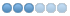# Write the exchange code?

• cry1004
• Topic Author
• Offline
• Senior Member
•7 months 2 weeks ago #9413 by cry1004
Write the exchange code? was created by cry1004
In the game I'm making, if you kill a monster, you don't pay money right away and drop bones.
The goal is to randomly change the currency exchange price at a specific time.

The following code calculates the sum of bones and coins and exchanges them.

When exchanging the maximum number of bones and the maximum number of coins, I want to make sure that the number does not overflow, but it seems to be complicated to make, so now I compare the number by subtracting one type from the total.

However, there may be a problem when the rate to be exchanged later changes over time.

For example, when you exchange bones for coins at the current time, if you exchange them for 120%, multiply the number of bones you have by 120%, and the calculated value should not exceed the number of coins you can hold.

If the exchange coin exceeds the maximum number of bones, I would like to inform you that only a few bones can be exchanged.

```SPECIAL(exchange)
{
int curr_bone, rest_bone;
long long int curr_coin;

curr_coin = (long long int)GET_BONE(ch)+(long long int)GET_COIN(ch);
if (CMD_IS("환전")) {
if (GET_COIN(ch) >= MAX_COIN) {
send_to_char(ch, "You can't have any more coins.\r\n");
return (TRUE);
}
if (GET_BONE(ch) <= 0) {
send_to_char(ch, "You don't have bones to exchange.\r\n");
return (TRUE);
}
if (curr_coin == MAX_COIN) {
curr_bone = GET_BONE(ch);

GET_COIN(ch) = MAX_COIN;
GET_BONE(ch) = 0;

send_to_char(ch, "%d bones were exchanged for coins.\r\n", curr_bone);
return (TRUE);
}
if (curr_coin > MAX_COIN) {
rest_bone = curr_coin - MAX_COIN;
curr_bone = GET_BONE(ch) - rest_bone;

GET_COIN(ch) = MAX_COIN;
GET_BONE(ch) = rest_bone;

send_to_char(ch, "%d bones were exchanged for coins.\r\n", curr_bone);
return (TRUE);
}
if (curr_coin < MAX_COIN) {
curr_bone = GET_BONE(ch);

GET_COIN(ch) = GET_COIN(ch) + GET_BONE(ch);
GET_BONE(ch) = 0;

send_to_char(ch, "%d  bones were exchanged for coins.\r\n", curr_bone);
return (TRUE);
}
}
return (FALSE);
}```

Please Log in or Create an account to join the conversation.

• thomas
•• Offline
• Administrator
•7 months 2 weeks ago #9423 by thomas
Replied by thomas on topic Write the exchange code?
Please login to view the answers
The following user(s) said Thank You: cry1004

Please Log in or Create an account to join the conversation.

Time to create page: 0.075 seconds